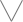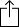C♭
D♭
E♭
F♭
G♭
A♭
B♭
Chord
Scale
Custom
3nps
CAGED
None
Notes
Degrees
Intervals
None

1
2
3
4
5
6
7
8
9
10
11
12
13
14
15
16
17
18
19
20
21
22
23
24
E
B
G
D
A
E
F
G
G
A
A
B
C
C
D
D
E
F
F
G
G
A
A
B
C
C
D
D
E
F
C
D
D
E
F
F
G
G
A
A
B
C
C
D
D
E
F
F
G
G
A
A
B
C
A
A
B
C
C
D
D
E
F
F
G
G
A
A
B
C
C
D
D
E
F
F
G
G
E
F
F
G
G
A
A
B
C
C
D
D
E
F
F
G
G
A
A
B
C
C
D
D
B
C
C
D
D
E
F
F
G
G
A
A
B
C
C
D
D
E
F
F
G
G
A
A
F
G
G
A
A
B
C
C
D
D
E
F
F
G
G
A
A
B
C
C
D
D
E
F

# Bb Oriental Scale for Guitar

Bb Oriental scale is a diatonic scale and is the 2nd mode in the set of Hungarian minor modes

B
C
D
E
F
G
A
BH
aug2
H
H
aug2
H
W
Play scaleand then